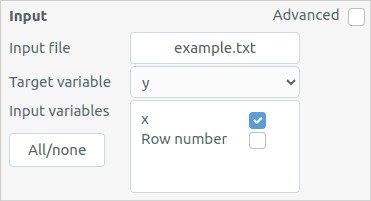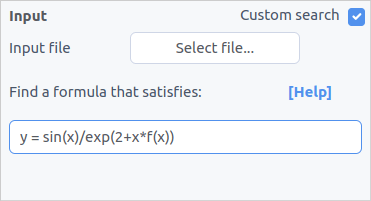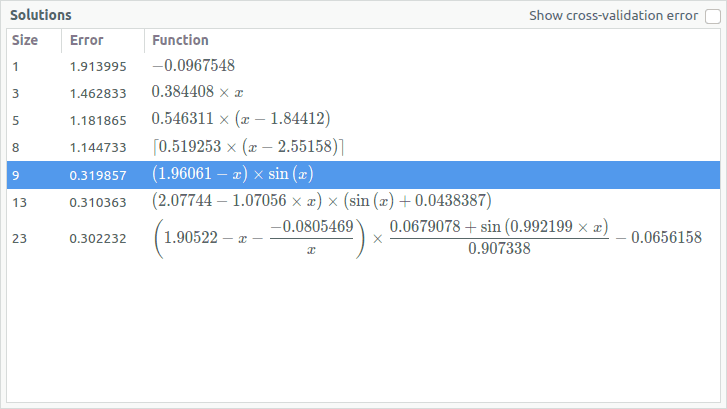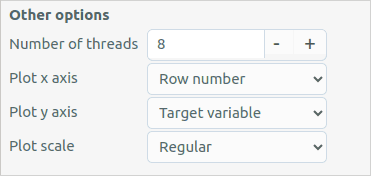## Introduction

TuringBot, named after great mathematician and computation pioneer Alan Turing, is a desktop software for Symbolic Regression. It uses a novel algorithm based on simulated annealing to discover mathematical formulas from values with unprecedented efficiency.

Data is read from TXT or CSV files, and the target and input columns can be selected from the interface. A variety of error metrics are available for the search, allowing the program to find formulas that solve both regression and classification problems.

The main features of TuringBot are the following:

• Pareto optimization: the software simultaneously tries to find the best formulas of all possible sizes. It will give you not only a single formula as output but a set of formulas of increasing complexity to choose from.
• Built-in cross-validation: allows you to easily rule out overfit solutions.
• Export solutions as Python, C/C++, or plain text.
• Multiprocessing.
• Written in a low-level programming language (no Python). Extremely fast.
• Has a command-line mode that allows you to automate the program.

## Input data format

Input files are selected on the interface by clicking on the "Input file" button, as shown below:The input file name must end in .txt or .csv, and the file must contain columns representing different variables separated by spaces, commas, semicolons, or tabs. Those values can be integers, floats, or floats in exponential notation (%d, %f, or %e), with decimal parts separated by a dot (1.61803 and not 1,61803).

Optionally, a header containing the variable names may be present in the first line of the file — in this case, those names will be used in the formulas instead of the default names, which are col1, col2, col3, etc. You also have the option of using the row number (1, 2, 3...) as an input variable, which is useful for time series data.

For example, the following is a valid input file:

```x y z
0.01231 0.99992 0.99985
0.23180 0.97325 0.94723
0.45128 0.89989 0.80980
0.67077 0.78334 0.61363
0.89026 0.62921 0.39591
1.00000 0.54030 0.29193
```

## Search optionsBefore starting the search, you can select a variety of search settings, including the error metric and the base functions that should be used in the optimization.

#### Error metrics

The available error metrics are:

 RMS error Root mean square error. Mean relative error Average of (absolute value of the error) / (absolute value of the target variable). Makes the convergence be in terms of relative error instead of absolute error. Classification accuracy (correct predictions) / (number of data points). Only useful for integer target values and classification problems. Mean error Average of (absolute value of the error). Similar to RMS error, but puts less emphasis on outliers. F-score 2*(precision*recall)/(precision+recall) by default when beta = 1. See here an image that explains what those two quantities are.This metric is useful for classification problems on highly imbalanced datasets, where the target variable is 0 for the majority of inputs and a positive integer on a few cases that need to be identified. If the classification is binary, then the categories can be 1 (relevant cases) and 0 (all other cases). Correlation coefficient Corresponds to the Pearson correlation coefficient. Useful for quickly getting the overall shape of the output right without much attention to scales. Hybrid (CC+RMS) The geometric mean between the correlation coefficient and the RMS error. This is a compromise between the attention to scale of the RMS metric and the speed of the CC metric. Maximum error The maximum absolute difference between the predictions of the model and the target variable. Maximum relative error The maximum of (absolute value of the error) / (absolute value of the target variable). Nash-Sutcliffe efficiency A metric that resembles the correlation coefficient and that is commonly used in hydrological applications. See the definition in this paper. Binary cross-entropy Used for solving binary classification problems in terms of probabilities. To use this metric, your target variable must contain two (and only two) classes represented by the numbers 0 and 1. Matthews Correlation Coefficient (MCC) Regarded to be one of the best classification metrics (see this paper), it combines true positives, true negatives, false positives, and false negatives into a single number between -1 and 1. To use this metric, your "negative" target variable must be represented by the number 0, and your "positive" variables must be represented by one or more positive integer numbers.

#### Base functions

The function names follow the conventions of the C math library. You can find their definitions on this page.

The only exceptions are the logical functions (logical_and(x, y), greater(x, y), etc) and the history functions (delay and moving average), which are defined internally in TuringBot.

The moving average of a variable is defined as its average value in the N rows before the present one. For instance, if the successive values for variable x are 1, 2, 3, 4, and 5, then the last moving average of x will be (2+3+4)/3. In some systems, this may be considered a moving average with a lag of 1.

The sizes of the base functions are defined as:

• Size 1: an input variable, sum, subtraction, and multiplication.
• Size 2: division.
• Size 3: abs(x), ceil(x), floor(x), and round(x).
• Size 4: all other functions.

#### Cross-validation

In the same box where the search options are selected, the cross-validation settings can also be selected. It is recommended to use cross-validation since that allows overfit models that are more complex than necessary to be discarded in a straightforward way.

The size of the train/test split can be selected from the menu. The default value, "No cross-validation", disables the cross-validation altogether. Two kinds of options are available: percentages like 80/20 and fixed-size training datasets like "100 rows" and "1000 rows". It is also possible to select how the training sample should be generated: if it should be a selection of random rows or if it should be the first rows in sequential order.

During the optimization, you can alternate between showing the errors for the training sample and the testing sample by clicking on the "Show cross-validation error" box on the upper right of the interface. With this, overfit solutions can be spotted in real-time.

By default, the program is configured to find formulas such that y = f(x1,x2,x3,...). But, in some cases, you might be interested in specific functional forms. Some examples could be:

• y = f()*x+f() (a line).
• y = f()*x*x + f()*x + f() (a parabola).
• y = f(x1,x2)*x1 + exp(f(x1)/4) + 2 (a formula with terms that should depend on specific variables).

This kind of search is possible with TuringBot's Advanced mode. To enable it, click the "Advanced" button and type your desired equation in the input box that will appear:The left side of the equation should be the desired variable, and the right side should be the formula that you are trying to find, with unknown terms denoted by f([variables]).

You can use in this equation any base function offered by the program, as well as numerical constants in integer, floating-point, or exponential notation (like 2, 3.14, or 2.35e-3).

The following conventions must be followed:

• Uknown terms must be denoted f([variables]). For a constant, use f(). For a function of x, use f(x). For a function of x and z, use f(x,z). Etc.
• For a function of all variables except one, you can use the "~" operator, which excludes variables. y = f(~y) will use all variables as input except for y. y = f(~y,~row) will use all variables except y and row.

During a custom search, the plot will show the left side of the equation as points and the right side of the equation as a line.

## The solutions box

The regression is started by clicking on the play button at the top of the interface. After that, the best solutions found so far will be shown in the "Solutions" box, as shown below:Each row corresponds to the best solution of a given size encountered so far. By clicking on a solution, it will be shown in the plot and its stats will be shown in the "Solution info" box. Larger solutions are only shown if they provide a better fit than all other smaller solutions.

We define the size of a solution as the sum of the sizes of the base functions that constitute it (see above).

In the Solutions box, you have the option of sorting the solutions by a balance between size and accuracy by clicking on the "Function" header, which will sort the solutions by (error)^2 * (size). By default, the solutions are sorted by size.

In the menu, you can find an option called "Set periodic output". There you can choose to enable two options for periodic output:

• Solutions: export formulas in the same format generated by "Export solutions as text".
• Predictions: export your original dataset along with the predictions of the models found by the program. Those columns will be called solution_N, where N is the complexity of each model.

The periodic output files are only saved if new solutions have been found, to not waste resources by saving the same files over and over again in long runs.

The solutions file generated by the periodic output option above can be loaded back into the program, allowing you to restart an optimization from a checkpoint. For that, start a new optimization and then choose the option "Load formulas from file" in the menu.

It is also possible to input your custom formulas into the program using this option. For that, generate a text file with one formula per row, making sure that the formulas do not contain any space characters, and load this file into the program.

## Plot settings

In the "Other options" part of the main tab you can at any time change your plot settings:For the y-axis, you can choose to plot your target variable, the residual error (difference between a solution and the target data), and the residual error as a percentage of the target data.

For the x-axis, you can choose the row number (1, 2, 3, ...) corresponding to that point, or any of your input variables. Additionally, you can also select the Observed option to see an Observed vs predicted plot.

The scales of the plot can be changed in the Plot scale menu, which allows you to choose between log x, log y, and log x and log y. The logarithm is calculated as a "symlog", allowing negative numbers to be visualized in a natural way.

In the "Advanced" tab of the interface, you can find information about how many formulas were generated in the current optimization, how long the optimization has been running, and how many formulas are being tried per second. A log message is also generated every time a new solution is encountered so that you can keep track of progress.

A few specialized search settings are also available on this tab:

• Maximum formula size: by default, the complexity of formulas is prevented from becoming larger than 60. With this option, you can allow the program to generate larger formulas, which makes the optimization slower but may be useful in some cases.
• Maximum history size: only used if one of the history functions is enabled. Sets the maximum length of those functions.
• F-score beta parameter: when left at the default value of 1, the F-score metric corresponds to the F1-metric. Values of beta lower than 1 favor precision over recall.
• Random seed for train/test split generation: if a value >= 0 is set, this value will be used as a seed, resulting in the same split every time a new search is started with the same dataset. When the parameter is set to -1, a different random split will be generated each time.
• Normalize the dataset: for each variable, subtract the average and divide by the standard deviation before starting the search. This can speed up the search a lot if your input variables are large. Note that the "sample standard deviation" is used, where the denominator is N-1 instead of N for smaller bias: link.
• Target variable in history functions: allows you to choose whether your target variable can be used in the history functions if one or more of these are enabled.
• Force solutions to include all variables: allows you to discard solutions that do not feature all input variables.
• Bound search mode: this advanced search mode allows you to discover formulas that are upper or lower bounds for the desired variable.

## Command-line usage

TuringBot is also a console application that can be executed in a fully automated and customizable way. The general usage is the following:

```TuringBot - Symbolic Regression Software

usage: turingbot [--help] INPUT_FILE [SETTINGS_FILE]
[--outfile FILENAME]
[--predictions-file FILENAME]
[--formulas-file FILENAME]

required arguments:
INPUT_FILE                    the full path to your input file:
use /foo/bar/file.txt
not ./file.txt or file.txt

optional arguments:
SETTINGS_FILE                 the full path to the settings file to use
for this optimization
--help                        show this help message
--outfile FILENAME            write the best formulas found so far
to this file
--predictions-file FILENAME   write the predictions obtained from the best
formulas found so far to this file
--formulas-file FILENAME      load seed formulas from this file.
the file generated by --outfile can be
later used as input here
the total number available in your system
```

If no configuration file is provided, the program will use the last column in the input file as the target variable and all other columns as input variables.

The best formulas found so far will be written to the terminal every 1 second. If you set an output file with the --outfile option, those formulas will also be regularly saved to the output file.

Note that to run the command above on Windows you have to first cd to the installation directory and then run with .\TuringBot.exe:

```cd C:\Program Files (x86)\TuringBot\TuringBot.exe
.\TuringBot.exe INPUT_FILE```

#### Settings file

The search can be fully customized by providing the program with a settings file. Here is an example:

```search_metric = 4 # Search metric. 1: Mean relative error, 2: Classification accuracy, 3: Mean error, 4: RMS error, 5:, F-score, 6: Correlation coefficient, 7: Hybrid (CC+RMS), 8: Maximum error, 9: Maximum relative error, 10: Nash-Sutcliffe efficiency
train_test_split = -1 # Train/test split. -1: No cross-validation. Valid options are: 50, 60, 70, 75, 80, 100, 1000, 10000, 100000
test_sample = 1 # Test sample. 1: Chosen randomly, 2: The last points
train_test_seed = -1 # Random seed for train/test split generation when the test sample is chosen randomly.
integer_constants = 0 # Integer constants only. 0: Disabled, 1: Enabled
bound_search_mode = 0 # Bound search mode. 0: Deactivated, 1: Lower bound search, 2: Upper bound search
maximum_formula_complexity = 60 # Maximum formula complexity.
history_size = 20 # History size.
fscore_beta = 1 # F-score beta parameter.
allow_target_delay = 1 # Allow the target variable in the lag functions? 0: No, 1: Yes
force_all_variables = 0 # Force solutions to include all input variables? 0: No, 1: Yes
custom_formula =  # Custom formula for the search. If empty, the program will try to find the last column as a function of the remaining ones.
allowed_functions = + * / pow fmod sin cos tan asin acos atan exp log log2 log10 sqrt sinh cosh tanh asinh acosh atanh abs floor ceil round sign tgamma lgamma erf # Allowed functions.
```

Settings are changed by modifying the values after the = characters. The comments after # characters are ignored. The allowed functions are set by directly providing their names to the allowed_functions variable, separated by spaces.

The order of the parameters inside the settings file does not matter.

A convenient way of generating a settings file is to set things as you like them in the graphical interface, and then simply export the settings from the menu using the "Save settings" option:#### Output

A typical terminal output of TuringBot is the following:

```Formulas generated: 1135108
Size   Error       Function
1      177813      186275.6979035278
3      7890.39     11.75045370574789*x
5      6895.25     11.93943363494786*(-472.8408126495318+x)
7      980.126     lgamma(1.22706776648686*x)
8      674.279     x*(0.6868942922296394+asinh(x))
9      240.168     1.062116924609507*acosh(x)*x
11     147.484     1.063188751909768*acosh(x)*(-34.51291396937853+x)
```

The first line reports how many formulas were attempted so far.

The next lines contain the formulas as well as their corresponding sizes and errors.

## Running TuringBot from Python

When you install TuringBot, you also receive a small Python library designed to make it very easy to call the software from within Python.

Below we provide examples of usage for each OS, but the basic idea is that this library provides a simulation class:

`sim = tb.simulation()`

This class has a start_process method that starts TuringBot in the background:

`sim.start_process(path, input_file, threads=4, config=config_file)`

The parameters that you see are:

• path (obligatory): the path to the TuringBot executable.
• input_file (obligatory): the path to the input file. By default, the last column will be set as the target variable, but you can fully customize the search by using a configuration file.
• threads=4 (optional): the number of threads that you want the program to use.
• config=config_file (optional): the path to the configuration file.

Once a simulation is started, you can refresh the current formulas with:

`sim.refresh_functions()`

and then access the formulas in the form of a list with:

`sim.functions`

You can also find general information about the number of formulas tried so far as well as error messages with:

`sim.info`

To finish a simulation and kill the TuringBot process, you should call

`sim.terminate_process()`

#### Windows

An example of usage of TuringBot's Python library on Windows is the following.

Note: please make sure that you have Python version 3.9.1 or greater installed for the code below to work properly on Windows.

```import sys
sys.path.insert(1, r'C:\Program Files (x86)\TuringBot')

import turingbot as tb
import time

path = r'C:\Program Files (x86)\TuringBot\TuringBot.exe'
input_file = r'C:\Users\user\Desktop\input.txt'
config_file = r'C:\Users\user\Desktop\settings.cfg'

sim = tb.simulation()

time.sleep(10)

sim.refresh_functions()
print(*sim.functions, sep='\n')
print(sim.info)

sim.terminate_process()
```

#### Linux

An example of usage of TuringBot's Python library on Linux is the following:

```import sys
sys.path.insert(1, '/usr/share/turingbot')

import turingbot as tb
import time

path = r'/usr/bin/turingbot'
input_file = r'/home/user/input.txt'
config_file = r'/home/user/settings.cfg'

sim = tb.simulation()

time.sleep(10)

sim.refresh_functions()
print(*sim.functions, sep='\n')
print(sim.info)

sim.terminate_process()
```

#### macOS

An example of usage of TuringBot's Python library on macOS is the following:

```import sys
sys.path.insert(1, '/Applications/TuringBot.app/Contents/Resources/')

import turingbot as tb
import time

path = r'/Applications/TuringBot.app/Contents/MacOS/turingbot-bin'
input_file = r'/Users/user/Desktop/input.txt'
config_file = r'/Users/user/Desktop/settings.cfg'

sim = tb.simulation()